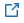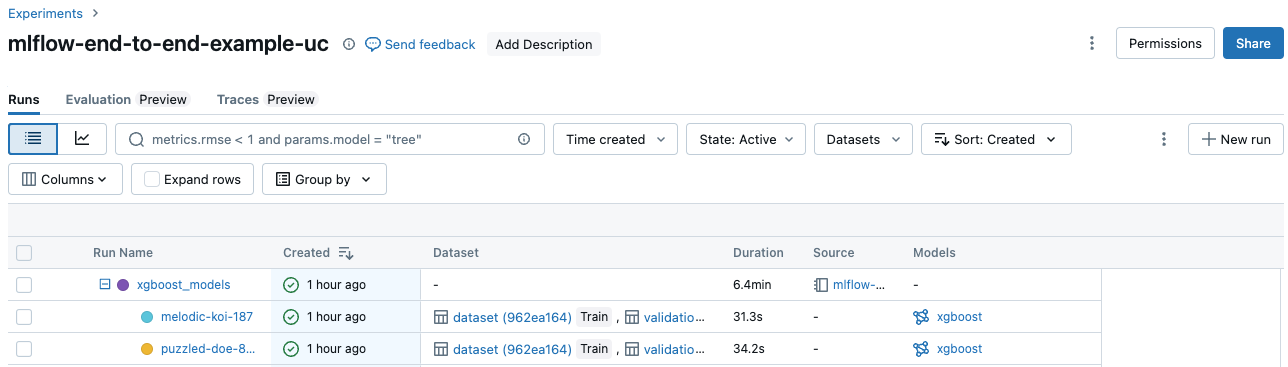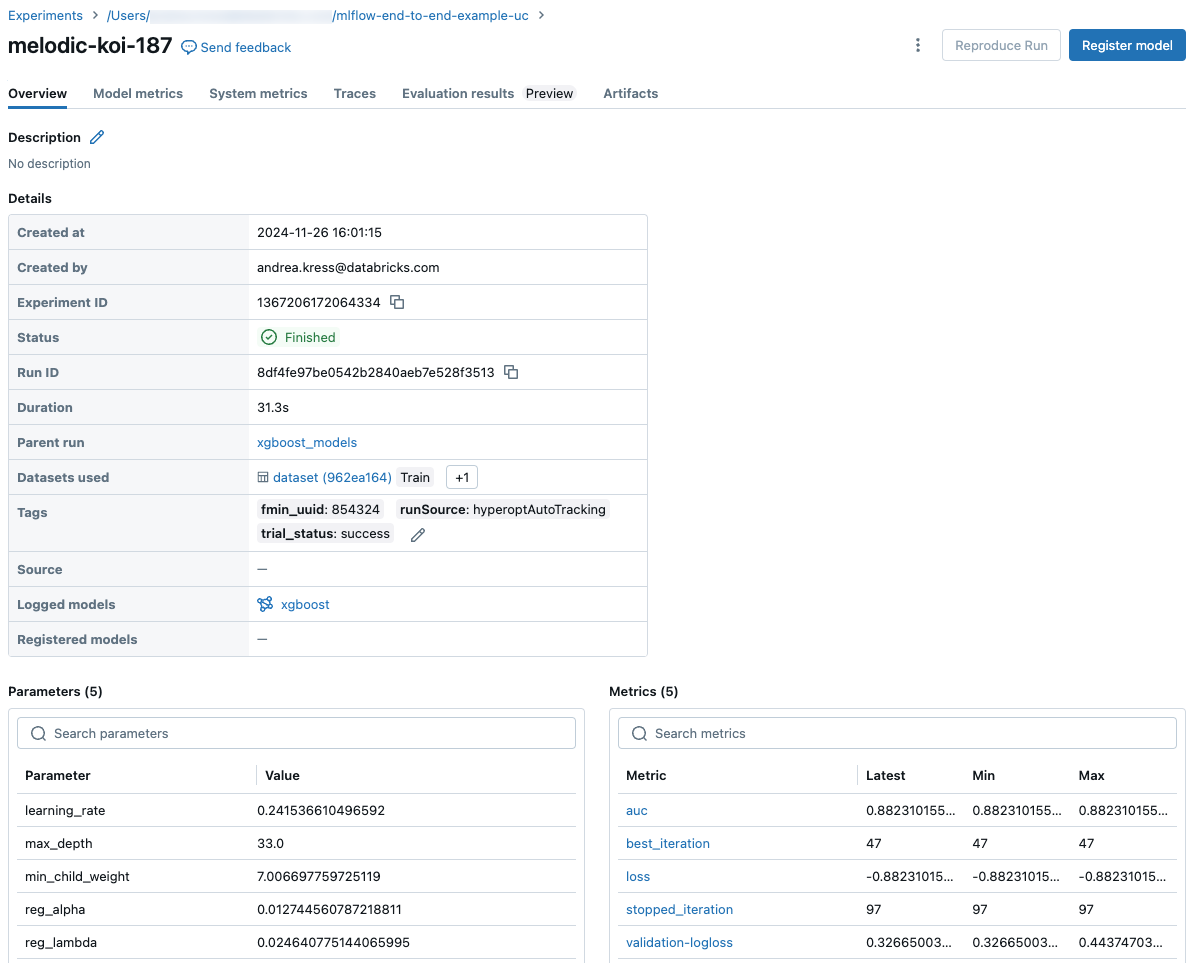# Quick start Python

MLflow is an open source platform for managing the end-to-end machine learning lifecycle. MLflow provides simple APIs for logging metrics (for example, model loss), parameters (for example, learning rate), and fitted models, making it easy to analyze training results or deploy models later on.

## Install MLflow

If you’re using Databricks Runtime for Machine Learning, MLflow is already installed. Otherwise, install the MLflow package from PyPI.

## Automatically log training runs to MLflow

MLflow provides `mlflow.<framework>.autolog()` APIs to automatically log training code written in many ML frameworks. You can call this API before running training code to log model-specific metrics, parameters, and model artifacts.

```# Also autoinstruments tf.keras
import mlflow.tensorflow
mlflow.tensorflow.autolog()
```
```# Use import mlflow.tensorflow and mlflow.tensorflow.autolog() if using tf.keras
import mlflow.keras
mlflow.keras.autolog()
```
```import mlflow.xgboost
mlflow.xgboost.autolog()
```
```import mlflow.lightgbm
mlflow.lightgbm.autolog()
```
```import mlflow.sklearn
mlflow.sklearn.autolog()
```

If performing tuning with `pyspark.ml`, metrics and models are automatically logged to MLflow. See Apache Spark MLlib and automated MLflow tracking

## View results

After executing your machine learning code, you can view results in the Experiment Runs sidebar:

1. Click the Experiment iconin the notebook context bar. The Experiments Runs sidebar displays. In the sidebar, you can view the run’s parameters and metrics:2. Click the External Link iconin the Experiment Runs context bar to view the experiment:3. In the experiment, click a date:The run details display:4. In the experiment, click a source:The notebook revision used in the run displays:## Track additional metrics, parameters, and models

You can log additional information by directly invoking the MLflow Tracking logging APIs.

• Numerical metrics:

```import mlflow
mlflow.log_metric("accuracy", 0.9)
```
• Training parameters:

```import mlflow
mlflow.log_param("learning_rate", 0.001)
```
• Models:

```import mlflow.sklearn
mlflow.sklearn.log_model(model, "myModel")
```
```import mlflow.spark
mlflow.spark.log_model(model, "myModel")
```
```import mlflow.xgboost
mlflow.xgboost.log_model(model, "myModel")
```
```import mlflow.tensorflow
mlflow.tensorflow.log_model(model, "myModel")
```
```import mlflow.keras
mlflow.keras.log_model(model, "myModel")
```
```import mlflow.pytorch
mlflow.pytorch.log_model(model, "myModel")
```
```import mlflow.spacy
mlflow.spacy.log_model(model, "myModel")
```
• Other artifacts (files):

```import mlflow
mlflow.log_artifact("/tmp/my-file", "myArtifactPath")
```

## Example notebooks

### Requirements

Databricks Runtime 6.4 or above or Databricks Runtime 6.4 ML or above.

### Notebooks

The recommended way to get started using MLflow tracking with Python is to use the MLflow `autolog()` API. With MLflow’s autologging capabilities, a single line of code automatically logs the resulting model, the parameters used to create the model, and a model score. The following notebook shows you how to set up a run using autologging.

#### MLflow Autologging Quick Start Python notebook

If you need more control over the metrics logged for each training run, or want to log additional artifacts such as tables or plots, you can use the MLflow logging API functions demonstrated in the following notebook.